Balbharati solutions for Mathematics and Statistics 1 (Arts and Science) 12th Standard HSC Maharashtra State Board chapter 1 - Mathematical Logic [Latest edition]

Chapters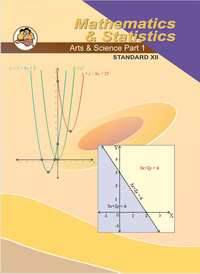Chapter 1: Mathematical Logic

Exercise 1.1Exercise 1.2Exercise 1.3Exercise 1.4Exercise 1.5Miscellaneous Exercise 1
Exercise 1.1 [Pages 6 - 8]

Balbharati solutions for Mathematics and Statistics 1 (Arts and Science) 12th Standard HSC Maharashtra State Board Chapter 1 Mathematical Logic Exercise 1.1 [Pages 6 - 8]

Exercise 1.1 | Q 1.01 | Page 6

State which of the following is the statement. Justify. In case of a statement, state its truth value.

5 + 4 = 13

Exercise 1.1 | Q 1.02 | Page 6

State which of the following is the statement. Justify. In case of a statement, state its truth value.

x – 3 = 14

Exercise 1.1 | Q 1.03 | Page 6

State which of the following is the statement. Justify. In case of a statement, state its truth value.

Close the door.

Exercise 1.1 | Q 1.04 | Page 6

State which of the following is the statement. Justify. In case of a statement, state its truth value.

Zero is a complex number.

Exercise 1.1 | Q 1.05 | Page 6

State which of the following is the statement. Justify. In case of a statement, state its truth value.

Exercise 1.1 | Q 1.06 | Page 6

State which of the following is the statement. Justify. In case of a statement, state its truth value.

Congruent triangles are similar.

Exercise 1.1 | Q 1.07 | Page 6

State which of the following is the statement. Justify. In case of a statement, state its truth value.

x2 = x

Exercise 1.1 | Q 1.08 | Page 8

State which of the following is the statement. Justify. In case of a statement, state its truth value.

A quadratic equation cannot have more than two roots.

Exercise 1.1 | Q 1.09 | Page 7

State which of the following is the statement. Justify. In case of a statement, state its truth value.

Do you like Mathematics?

Exercise 1.1 | Q 1.1 | Page 7

State which of the following is the statement. Justify. In case of a statement, state its truth value.

The sunsets in the west

Exercise 1.1 | Q 1.11 | Page 7

State which of the following is the statement. Justify. In case of a statement, state its truth value.

All real numbers are whole numbers.

Exercise 1.1 | Q 1.12 | Page 7

State which of the following is the statement. Justify. In case of a statement, state its truth value.

Can you speak in Marathi?

Exercise 1.1 | Q 1.13 | Page 7

State which of the following is the statement. Justify. In case of a statement, state its truth value.

x2 – 6x – 7 = 0, when x = 7

Exercise 1.1 | Q 1.14 | Page 7

State which of the following is the statement. Justify. In case of a statement, state its truth value.

The sum of cube roots of unity is zero.

Exercise 1.1 | Q 1.15 | Page 7

State which of the following is the statement. Justify. In case of a statement, state its truth value.

It rains heavily.

Exercise 1.1 | Q 2.1 | Page 7

Write the following compound statement symbolically.

Nagpur is in Maharashtra and Chennai is in Tamil Nadu.

Exercise 1.1 | Q 2.3 | Page 7

Write the following compound statement symbolically.

The angle is right angle if and only if it is of measure 90°.

Exercise 1.1 | Q 2.4 | Page 7

Write the following compound statement symbolically.

Angle is neither acute nor obtuse.

Exercise 1.1 | Q 2.5 | Page 7

Write the following compound statement symbolically.

If Δ ABC is right-angled at B, then m∠A + m∠C = 90°

Exercise 1.1 | Q 2.6 | Page 7

Write the following compound statement symbolically.

Hima Das wins gold medal if and only if she runs fast.

Exercise 1.1 | Q 2.7 | Page 7

Write the following compound statement symbolically.

x is not irrational number but is a square of an integer.

Exercise 1.1 | Q 3.1 | Page 7

Write the truth values of the following.

4 is odd or 1 is prime.

Exercise 1.1 | Q 3.2 | Page 7

Write the truth values of the following.

64 is a perfect square and 46 is a prime number.

Exercise 1.1 | Q 3.3 | Page 7

Write the truth values of the following.

5 is a prime number and 7 divides 94.

Exercise 1.1 | Q 3.4 | Page 7

Write the truth values of the following.

It is not true that 5−3i is a real number.

Exercise 1.1 | Q 3.5 | Page 7

Write the truth value of the following.

If 3 × 5 = 8 then 3 + 5 = 15.

Exercise 1.1 | Q 3.6 | Page 7

Write the truth value of the following.

Milk is white if and only if sky is blue.

Exercise 1.1 | Q 3.7 | Page 7

Write the truth values of the following.

24 is a composite number or 17 is a prime number.

Exercise 1.1 | Q 4.1 | Page 7

If the statement p, q are true statement and r, s are false statement then determine the truth value of the following:

p ∨ (q ∧ r)

Exercise 1.1 | Q 4.2 | Page 7

If the statement p, q are true statement and r, s are false statement then determine the truth value of the following:

(p → q) ∨ (r → s)

Exercise 1.1 | Q 4.3 | Page 7

If the statement p, q are true statement and r, s are false statement then determine the truth value of the following:

(q ∧ r) ∨ (∼ p ∧ s)

Exercise 1.1 | Q 4.4 | Page 7

If the statement p, q are true statement and r, s are false statement then determine the truth value of the following:

(p → q) ∧ ∼ r

Exercise 1.1 | Q 4.5 | Page 7

If the statement p, q are true statement and r, s are false statement then determine the truth value of the following:

(∼ r ↔ p) → ∼ q

Exercise 1.1 | Q 4.6 | Page 7

If the statement p, q are true statement and r, s are false statement then determine the truth value of the following:

[∼ p ∧ (∼ q ∧ r)] ∨ [(q ∧ r) ∨ (p ∧ r)]

Exercise 1.1 | Q 4.7 | Page 7

If the statement p, q are true statement and r, s are false statement then determine the truth value of the following:

[(∼ p ∧ q) ∧ ∼ r] ∨ [(q → p) → (∼ s ∨ r)]

Exercise 1.1 | Q 4.8 | Page 7

If the statement p, q are true statement and r, s are false statement then determine the truth value of the following:

∼ [(∼ p ∧ r) ∨ (s → ∼ q)] ↔ (p ∧ r)

Exercise 1.1 | Q 5.1 | Page 7

Write the negation of the following.

Exercise 1.1 | Q 5.2 | Page 7

Write the negation of the following.

3 is not a root of the equation x2 + 3x – 18 = 0

Exercise 1.1 | Q 5.3 | Page 7

Write the negation of the following.

sqrt2 is a rational number.

Exercise 1.1 | Q 5.4 | Page 7

Write the negation of the following.

Polygon ABCDE is a pentagon.

Exercise 1.1 | Q 5.5 | Page 7

Write the negation of the following.

7 + 3 > 5
Exercise 1.2 [Page 13]

Balbharati solutions for Mathematics and Statistics 1 (Arts and Science) 12th Standard HSC Maharashtra State Board Chapter 1 Mathematical Logic Exercise 1.2 [Page 13]

Exercise 1.2 | Q 1.01 | Page 13

Construct the truth table of the following statement pattern.

[(p → q) ∧ q] → p

Exercise 1.2 | Q 1.02 | Page 13

Construct the truth table of the following statement pattern.

(p ∧ ∼q) ↔ (p → q)

Exercise 1.2 | Q 1.03 | Page 13

Construct the truth table of the following statement pattern.

(p ∧ q) ↔ (q ∨ r)

Exercise 1.2 | Q 1.04 | Page 13

Construct the truth table of the following statement pattern.

p → [∼ (q ∧ r)]

Exercise 1.2 | Q 1.05 | Page 13

Construct the truth table of the following statement pattern.

∼ p ∧ [(p ∨ ∼ q) ∧ q]

Exercise 1.2 | Q 1.06 | Page 13

Construct the truth table of the following statement pattern.

(∼ p → ∼ q) ∧ (∼ q → ∼ p)

Exercise 1.2 | Q 1.07 | Page 13

Construct the truth table of the following statement pattern.

(q → p) ∨ (∼ p ↔ q)

Exercise 1.2 | Q 1.08 | Page 13

Construct the truth table of the following statement pattern.

[p → (q → r)] ↔ [(p ∧ q) → r]

Exercise 1.2 | Q 1.09 | Page 13

Construct the truth table of the following statement pattern.

p → [∼ (q ∧ r)]

Exercise 1.2 | Q 1.1 | Page 13

Construct the truth table of the following statement pattern.

(p ∨ ∼ q) → (r ∧ p)

Exercise 1.2 | Q 2.01 | Page 13

Using truth table, prove that ~ p ∧ q ≡ (p ∨ q) ∧ ~ p

Exercise 1.2 | Q 2.02 | Page 13

Using the truth table prove the following logical equivalence.

∼ (p ∨ q) ∨ (∼ p ∧ q) ≡ ∼ p

Exercise 1.2 | Q 2.03 | Page 13

Using the truth table prove the following logical equivalence.

p ↔ q ≡ ∼ [(p ∨ q) ∧ ∼ (p ∧ q)]

Exercise 1.2 | Q 2.04 | Page 13

Using the truth table prove the following logical equivalence.

p → (q → p) ≡ ∼ p → (p → q)

Exercise 1.2 | Q 2.05 | Page 13

Using the truth table prove the following logical equivalence.

(p ∨ q) → r ≡ (p → r) ∧ (q → r)

Exercise 1.2 | Q 2.06 | Page 13

Using the truth table prove the following logical equivalence.

p → (q ∧ r) ≡ (p → q) ∧ (p → r)

Exercise 1.2 | Q 2.07 | Page 13

Using the truth table prove the following logical equivalence.

p → (q ∧ r) ≡ (p ∧ q) (p → r)

Exercise 1.2 | Q 2.08 | Page 13

Using the truth table prove the following logical equivalence.

p ∧ (q ∨ r) ≡ (p ∧ q) ∨ (p ∧ r)

Exercise 1.2 | Q 2.09 | Page 13

Using the truth table prove the following logical equivalence.

[∼ (p ∨ q) ∨ (p ∨ q)] ∧ r ≡ r

Exercise 1.2 | Q 2.1 | Page 13

Using the truth table proves the following logical equivalence.

∼ (p ↔ q) ≡ (p ∧ ∼ q) ∨ (q ∧ ∼ p)

Exercise 1.2 | Q 3.01 | Page 13

Examine whether the following statement pattern is a tautology or a contradiction or a contingency.

(p ∧ q) → (q ∨ p)

• Tautology

• Contingency

Exercise 1.2 | Q 3.02 | Page 13

Examine whether the following statement pattern is a tautology or a contradiction or a contingency.

(p → q) ↔ (∼ p ∨ q)

Exercise 1.2 | Q 3.03 | Page 13

Discuss the statement pattern, using truth table : ~(~p ∧ ~q) v q

Exercise 1.2 | Q 3.04 | Page 13

Examine whether the following logical statement pattern is a tautology, contradiction, or contingency.
[(p → q) ∧ q] → p

Exercise 1.2 | Q 3.05 | Page 13

Examine whether the following statement pattern is a tautology or a contradiction or a contingency.

[(p → q) ∧ ∼ q] → ∼ p

Exercise 1.2 | Q 3.06 | Page 13

Examine whether the following statement pattern is a tautology or a contradiction or a contingency.

(p ↔ q) ∧ (p → ∼ q)

Exercise 1.2 | Q 3.07 | Page 13

Examine whether the following statement pattern is a tautology or a contradiction or a contingency.

∼ (∼ q ∧ p) ∧ q

Exercise 1.2 | Q 3.08 | Page 13

Examine whether the following statement pattern is a tautology or a contradiction or a contingency.

(p ∧ ∼ q) ↔ (p → q)

Exercise 1.2 | Q 3.09 | Page 13

Examine whether the following statement pattern is a tautology or a contradiction or a contingency.

(∼ p → q) ∧ (p ∧ r)

Exercise 1.2 | Q 3.1 | Page 13

Examine whether the following statement pattern is a tautology or a contradiction or a contingency.

[p → (∼ q ∨ r)] ↔ ∼ [p → (q → r)]

Exercise 1.3 [Pages 17 - 18]

Balbharati solutions for Mathematics and Statistics 1 (Arts and Science) 12th Standard HSC Maharashtra State Board Chapter 1 Mathematical Logic Exercise 1.3 [Pages 17 - 18]

Exercise 1.3 | Q 1.1 | Page 17

If A = {3, 5, 7, 9, 11, 12}, determine the truth value of the following.

∃ x ∈ A such that x – 8 = 1

Exercise 1.3 | Q 1.2 | Page 17

If A = {3, 5, 7, 9, 11, 12}, determine the truth value of the following.

∀ x ∈ A, x2 + x is an even number

Exercise 1.3 | Q 1.3 | Page 17

If A = {3, 5, 7, 9, 11, 12}, determine the truth value of the following.

∃ x ∈ A such that x2 < 0

Exercise 1.3 | Q 1.4 | Page 17

If A = {3, 5, 7, 9, 11, 12}, determine the truth value of the following.

∀ x ∈ A, x is an even number

Exercise 1.3 | Q 1.5 | Page 17

If A = {3, 5, 7, 9, 11, 12}, determine the truth value of the following.

∃ x ∈ A such that 3x + 8 > 40

Exercise 1.3 | Q 1.6 | Page 17

If A = {3, 5, 7, 9, 11, 12}, determine the truth value of the following.

∀ x ∈ A, 2x + 9 > 14

Exercise 1.3 | Q 2.01 | Page 17

Write the dual of the following.

p ∨ (q ∧ r)

Exercise 1.3 | Q 2.02 | Page 17

Write the dual of the following.

p ∧ (q ∧ r)

Exercise 1.3 | Q 2.03 | Page 17

Write the dual of the following.

(p ∨ q) ∧ (r ∨ s)

Exercise 1.3 | Q 2.04 | Page 17

Write the dual of the following.

p ∧ ∼ q

Exercise 1.3 | Q 2.05 | Page 17

Write the dual of the following.

(∼ p ∨ q) ∧ (∼ r ∧ s)

Exercise 1.3 | Q 2.06 | Page 17

Write the dual of the following.

∼ p ∧ (∼ q ∧ (p ∨ q) ∧ ∼ r)

Exercise 1.3 | Q 2.07 | Page 17

Write the dual of the following.

[∼ (p ∨ q)] ∧ [p ∨ ∼ (q ∧ ∼ s)]

Exercise 1.3 | Q 2.08 | Page 17

Write the dual of the following.

c ∨ {p ∧ (q ∨ r)}

Exercise 1.3 | Q 2.09 | Page 17

Write the dual of the following.

∼ p ∨ (q ∧ r) ∧ t

Exercise 1.3 | Q 2.1 | Page 17

Write the dual of the following.

(p ∨ q) ∨ c

Exercise 1.3 | Q 3.1 | Page 18

Write the negation of the following.

x + 8 > 11 or y – 3 = 6

Exercise 1.3 | Q 3.2 | Page 18

Write the negation of the following.

11 < 15 and 25 > 20

Exercise 1.3 | Q 3.3 | Page 18

Write the negation of the following.

Quadrilateral is a square if and only if it is a rhombus.

Exercise 1.3 | Q 3.4 | Page 18

Write the negation of the following.

It is cold and raining.

Exercise 1.3 | Q 3.5 | Page 18

Write the negation of the following.

If it is raining then we will go and play football.

Exercise 1.3 | Q 3.6 | Page 18

Write the negation of the following.

sqrt2 is a rational number.

Exercise 1.3 | Q 3.7 | Page 18

Write the negation of the following.

All-natural numbers are whole numbers.

Exercise 1.3 | Q 3.8 | Page 18

Write the negation of the following.

∀ n ∈ N, n2 + n + 2 is divisible by 4.

Exercise 1.3 | Q 3.9 | Page 18

Write the negation of the following.

∃ x ∈ N such that x – 17 < 20

Exercise 1.3 | Q 4.1 | Page 18

Write converse, inverse and contrapositive of the following statement.

If x < y then x2 < y2 (x, y ∈ R)

Exercise 1.3 | Q 4.2 | Page 18

Write converse, inverse and contrapositive of the following statement.

A family becomes literate if the woman in it is literate.

Exercise 1.3 | Q 4.3 | Page 18

Write converse, inverse and contrapositive of the following statement.

If surface area decreases then pressure increases.

Exercise 1.3 | Q 4.4 | Page 18

Write converse, inverse and contrapositive of the following statement.

If voltage increases then current decreases.

Exercise 1.4 [Page 21]

Balbharati solutions for Mathematics and Statistics 1 (Arts and Science) 12th Standard HSC Maharashtra State Board Chapter 1 Mathematical Logic Exercise 1.4 [Page 21]

Exercise 1.4 | Q 1.1 | Page 21

Using the rule of negation write the negation of the following with justification.

∼ q → p

Exercise 1.4 | Q 1.2 | Page 21

Using the rule of negation write the negation of the following with justification.

p ∧ ∼ q

Exercise 1.4 | Q 1.3 | Page 21

Using the rule of negation write the negation of the following with justification.

p ∨ ∼ q

Exercise 1.4 | Q 1.4 | Page 21

Using the rule of negation write the negation of the following with justification.

(p ∨ ∼ q) ∧ r

Exercise 1.4 | Q 1.5 | Page 21

Using the rule of negation write the negation of the following with justification.

p → (p ∨ ∼ q)

Exercise 1.4 | Q 1.6 | Page 21

Using the rule of negation write the negation of the following with justification.

∼ (p ∧ q) ∨ (p ∨ ∼ q)

Exercise 1.4 | Q 1.7 | Page 21

Using the rule of negation write the negation of the following with justification.

(p ∨ ∼ q) → (p ∧ ∼ q)

Exercise 1.4 | Q 1.8 | Page 21

Using the rule of negation write the negation of the following with justification.

(∼ p ∨ ∼ q) ∨ (p ∧ ∼ q)

Exercise 1.4 | Q 2.1 | Page 21

Rewrite the following statement without using if ...... then.

If a man is a judge then he is honest.

Exercise 1.4 | Q 2.2 | Page 21

Rewrite the following statement without using if ...... then.

It 2 is a rational number then sqrt2 is irrational number.

Exercise 1.4 | Q 2.3 | Page 21

Rewrite the following statement without using if ...... then.

It f(2) = 0 then f(x) is divisible by (x – 2).

Exercise 1.4 | Q 3.1 | Page 21

Without using the truth table show that P ↔ q ≡ (p ∧ q) ∨ (~ p ∧ ~ q)

Exercise 1.4 | Q 3.2 | Page 21

Without using truth table prove that:

(p ∨ q) ∧ (p ∨ ∼ q) ≡ p

Exercise 1.4 | Q 3.3 | Page 21

Without using truth table prove that:

(p ∧ q) ∨ (∼ p ∧ q) ∨ (p ∧ ∼ q) ≡ p ∨ q

Exercise 1.4 | Q 3.4 | Page 21

Without using truth table prove that:

∼ [(p ∨ ∼ q) → (p ∧ ∼ q)] ≡ (p ∨ ∼ q) ∧ (∼ p ∨ q)

Exercise 1.5 [Pages 29 - 30]

Balbharati solutions for Mathematics and Statistics 1 (Arts and Science) 12th Standard HSC Maharashtra State Board Chapter 1 Mathematical Logic Exercise 1.5 [Pages 29 - 30]

Exercise 1.5 | Q 1.1 | Page 29

Express the following circuit in the symbolic form of logic and writ the input-output table.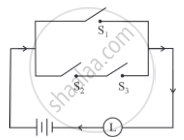Exercise 1.5 | Q 1.2 | Page 29

Express the following circuit in the symbolic form of logic and writ the input-output table.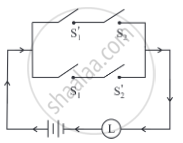Exercise 1.5 | Q 1.3 | Page 29

Express the following circuit in the symbolic form of logic and writ the input-output table.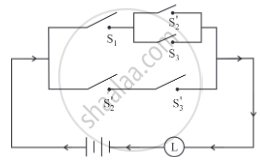Exercise 1.5 | Q 1.4 | Page 29

Express the following circuit in the symbolic form of logic and writ the input-output table.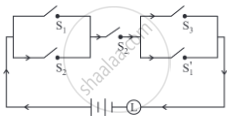Exercise 1.5 | Q 1.5 | Page 29

Express the following circuit in the symbolic form of logic and writ the input-output table.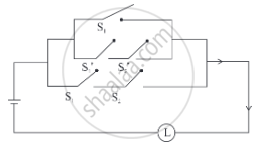Exercise 1.5 | Q 1.6 | Page 29

Express the following circuit in the symbolic form of logic and writ the input-output table.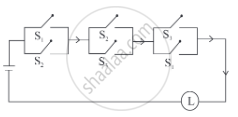Exercise 1.5 | Q 2.1 | Page 30

Construct the switching circuit of the following:

(∼ p ∧ q) ∨ (p ∧ ∼ r)

Exercise 1.5 | Q 2.2 | Page 30

Construct the switching circuit of the following:

(p ∧ q) ∨ [∼ p ∧ (∼ q ∨ p ∨ r)]

Exercise 1.5 | Q 2.3 | Page 30

Construct the switching circuit of the following:

(p ∧ r) ∨ (∼ q ∧ ∼ r)] ∧ (∼ p ∧ ∼ r)

Exercise 1.5 | Q 2.4 | Page 30

Construct the switching circuit of the following:

(p ∧ ∼ q ∧ r) ∨ [p ∧ (∼ q ∨ ∼ r)]

Exercise 1.5 | Q 2.5 | Page 30

Construct the switching circuit of the following:

p ∨ (∼ p) ∨ (∼ q) ∨ (p ∧ q)

Exercise 1.5 | Q 2.6 | Page 30

Construct the switching circuit of the following:

(p ∧ q) ∨ (∼ p) ∨ (p ∧ ∼ q)

Exercise 1.5 | Q 3.1 | Page 30

Give an alternative equivalent simple circuit for the following circuit: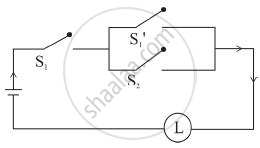Exercise 1.5 | Q 3.2 | Page 30

Give an alternative equivalent simple circuit for the following circuit: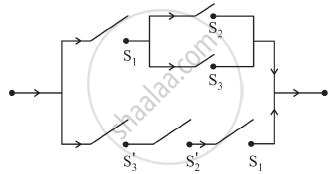Exercise 1.5 | Q 4.1 | Page 30

find the symbolic fom of the following switching circuit, construct its switching table and interpret it.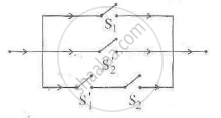Exercise 1.5 | Q 4.2 | Page 30

Write the symbolic form of the following switching circuit construct its switching table and interpret it.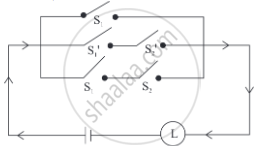Exercise 1.5 | Q 4.3 | Page 30

Write the symbolic form of the following switching circuit construct its switching table and interpret it.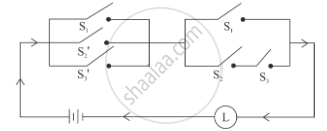Exercise 1.5 | Q 5.1 | Page 30

Obtain the simple logical expression of the following. Draw the corresponding switching circuit.

p ∨ (q ∧ ∼ q)

Exercise 1.5 | Q 5.2 | Page 30

Obtain the simple logical expression of the following. Draw the corresponding switching circuit.

(∼ p ∧ q) ∨ (∼ p ∧ ∼ q) ∨ (p ∧ ∼ q)

Exercise 1.5 | Q 5.3 | Page 30

Obtain the simple logical expression of the following. Draw the corresponding switching circuit.

[p ∨ ( ∼ q) ∨ (∼ r)] ∧ [p ∨ (q ∧ r)]

Exercise 1.5 | Q 5.4 | Page 30

Obtain the simple logical expression of the following. Draw the corresponding switching circuit.

(p ∧ q ∧ ∼ p) ∨ (∼ p ∧ q ∧ r) ∨ (p ∧ ∼ q ∧ r)

Miscellaneous Exercise 1 [Pages 32 - 35]

Balbharati solutions for Mathematics and Statistics 1 (Arts and Science) 12th Standard HSC Maharashtra State Board Chapter 1 Mathematical Logic Miscellaneous Exercise 1 [Pages 32 - 35]

Miscellaneous Exercise 1 | Q 1.1 | Page 32

Select and write the correct answer from the given alternative of the following question:

If p ∧ q is false and p ∨ q is true, then __________ is not true.

• p ∨ q

• p ↔ q

• ∼p ∨ ∼q

• q ∨ ∼p

Miscellaneous Exercise 1 | Q 1.2 | Page 32

Select and write the correct answer from the given alternative of the following question:

(p ∧ q) → r is logically equivalent to ________ .

• p → (q → r)

• (p ∧ q) → ∼r

• (∼p ∨ ∼q) → ∼r

• (p ∨ q) → r

Miscellaneous Exercise 1 | Q 1.3 | Page 32

Select and write the correct answer from the given alternative of the following question:

Inverse of statement pattern (p ∨ q) → (p ∧ q) is ________ .

• (p ∧ q) → (p ∨ q)

• ∼ (p ∨ q) → (p ∧ q)

• (∼p ∧ ∼q) → (∼p ∨ ∼q)

• (∼p ∨ ∼q) → (∼p ∧ ∼q)

Miscellaneous Exercise 1 | Q 1.4 | Page 32

Select and write the correct answer from the given alternative of the following question:

If p ∧ q is F, p → q is F then the truth values of p and q are ________.

• T, T

• T, F

• F, T

• F, F

Miscellaneous Exercise 1 | Q 1.5 | Page 32

Select and write the correct answer from the given alternative of the following question:

The negation of inverse of ∼p → q is ________.

• q ∧ p

• ∼p ∧ ∼q

• p ∧ q

• ∼q → ∼p

Miscellaneous Exercise 1 | Q 1.6 | Page 32

Select and write the correct answer from the given alternative of the following question:

The negation of p ∧ (q → r) is ________.

• ∼p ∧ (∼q → ∼r)

• p ∨ (∼q ∨ r)

• ∼p ∧ (∼q → ∼r)

• ∼p ∨ (∼q ∧ ∼r)

Miscellaneous Exercise 1 | Q 1.7 | Page 32

Select and write the correct answer from the given alternative of the following question:

If A = {1, 2, 3, 4, 5} then which of the following is not true?

• ∃ x ∈ A such that x + 3 = 8

• ∃ x ∈ A such that x + 2 < 9

• ∀ x ∈ A, x + 6 ≥ 9

• ∃ x ∈ A such that x + 6 < 10

Miscellaneous Exercise 1 | Q 2.1 | Page 33

Which of the following sentence is the statement in logic? Justify. Write down the truth value of the statement:

4! = 24.

Miscellaneous Exercise 1 | Q 2.2 | Page 33

Which of the following sentence is the statement in logic? Justify. Write down the truth value of the statement:

π is an irrational number.

Miscellaneous Exercise 1 | Q 2.3 | Page 33

Which of the following sentence is the statement in logic? Justify. Write down the truth value of the statement:

India is a country and Himalayas is a river.

Miscellaneous Exercise 1 | Q 2.4 | Page 33

Which of the following sentence is the statement in logic? Justify. Write down the truth value of the statement:

Please get me a glass of water.

Miscellaneous Exercise 1 | Q 2.5 | Page 33

Which of the following sentence is the statement in logic? Justify. Write down the truth value of the statement:

cos2θ − sin2θ = cos2θ for all θ∈R.

Miscellaneous Exercise 1 | Q 2.6 | Page 33

Which of the following sentence is the statement in logic? Justify. Write down the truth value of the statement:

If x is a whole number then x + 6 = 0.

Miscellaneous Exercise 1 | Q 3.1 | Page 33

Write the truth value of the following statement:

sqrt5 is an irrational but 3sqrt5 is a complex number.

Miscellaneous Exercise 1 | Q 3.2 | Page 33

Write the truth value of the following statement:

∀ n ∈ N, n2 + n is even number while n2 – n is an odd number.

Miscellaneous Exercise 1 | Q 3.3 | Page 33

Write the truth value of the following statement:

∃ n ∈ N such that n + 5 > 10.

Miscellaneous Exercise 1 | Q 3.4 | Page 33

Write the truth value of the following statement:

The square of any even number is odd or the cube of any odd number is odd.

Miscellaneous Exercise 1 | Q 3.5 | Page 33

Write the truth value of the following statement:

In ΔABC if all sides are equal then its all angles are equal.

Miscellaneous Exercise 1 | Q 3.6 | Page 33

Write the truth value of the following statement:

∀ n ∈ N, n + 6 > 8.

Miscellaneous Exercise 1 | Q 4.1 | Page 33

If A = {1, 2, 3, 4, 5, 6, 7, 8, 9}, determine the truth value of the following statement:

∃ x ∈ A such that x + 8 = 15

Miscellaneous Exercise 1 | Q 4.2 | Page 33

If A = {1, 2, 3, 4, 5, 6, 7, 8, 9}, determine the truth value of the following statement:

∀ x ∈ A, x + 5 < 12.

Miscellaneous Exercise 1 | Q 4.3 | Page 33

If A = {1, 2, 3, 4, 5, 6, 7, 8, 9}, determine the truth value of the following statement:

∃ x ∈ A, such that x + 7 ≥ 11.

Miscellaneous Exercise 1 | Q 4.4 | Page 33

If A = {1, 2, 3, 4, 5, 6, 7, 8, 9}, determine the truth value of the following statement:

∀ x ∈ A, 3x ≤ 25.

Miscellaneous Exercise 1 | Q 5.1 | Page 33

Write the negation of the following:

∀ n ∈ A, n + 7 > 6.

Miscellaneous Exercise 1 | Q 5.2 | Page 33

Write the negation of the following:

∃ x ∈ A, such that x + 9 ≤ 15.

Miscellaneous Exercise 1 | Q 5.3 | Page 33

Write the negation of the following:

Some triangles are equilateral triangle.

Miscellaneous Exercise 1 | Q 6.1 | Page 33

Construct the truth table of the following:

p → (q → p)

Miscellaneous Exercise 1 | Q 6.2 | Page 33

Construct the truth table of the following:

(∼p ∨ ∼q) ↔ [∼(p ∧ q)]

Miscellaneous Exercise 1 | Q 6.3 | Page 33

Construct the truth table of the following:

∼ (∼p ∧ ∼q) ∨ q

Miscellaneous Exercise 1 | Q 6.4 | Page 33

Construct the truth table of the following:

[(p ∧ q) ∨ r] ∧ [∼r ∨ (p ∧ q)]

Miscellaneous Exercise 1 | Q 6.5 | Page 33

Construct the truth table of the following:

[(∼p ∨ q) ∧ (q → r)] → (p → r)

Miscellaneous Exercise 1 | Q 7.1 | Page 33

Examine whether the following statement pattern is a tautology or a contradiction or a contingency.

[(p → q) ∧ ∼ q] → ∼ p

Miscellaneous Exercise 1 | Q 7.2 | Page 33

Determine whether the following statement pattern is a tautology, contradiction, or contingency:

[(p ∨ q) ∧ ∼p] ∧ ∼q

Miscellaneous Exercise 1 | Q 7.3 | Page 33

Determine whether the following statement pattern is a tautology, contradiction, or contingency:

(p → q) ∧ (p ∧ ∼q)

Miscellaneous Exercise 1 | Q 7.4 | Page 33

Determine whether the following statement pattern is a tautology, contradiction or contingency:

[p → (q → r)] ↔ [(p ∧ q) → r]

Miscellaneous Exercise 1 | Q 7.5 | Page 33

Determine whether the following statement pattern is a tautology, contradiction or contingency:

[(p ∧ (p → q)] → q

Miscellaneous Exercise 1 | Q 7.6 | Page 33

Determine whether the following statement pattern is a tautology, contradiction or contingency:

(p ∧ q) ∨ (∼p ∧ q) ∨ (p ∨ ∼q) ∨ (∼p ∧ ∼q)

Miscellaneous Exercise 1 | Q 7.7 | Page 33

Determine whether the following statement pattern is a tautology, contradiction or contingency:

[(p ∨ ∼q) ∨ (∼p ∧ q)] ∧ r

Miscellaneous Exercise 1 | Q 7.8 | Page 33

Determine whether the following statement pattern is a tautology, contradiction or contingency:

(p → q) ∨ (q → p)

Miscellaneous Exercise 1 | Q 8.1 | Page 34

Determine the truth values of p and q in the following case:

(p ∨ q) is T and (p ∧ q) is T

Miscellaneous Exercise 1 | Q 8.2 | Page 34

Determine the truth values of p and q in the following case:

(p ∨ q) is T and (p ∨ q) → q is F

Miscellaneous Exercise 1 | Q 8.3 | Page 34

Determine the truth values of p and q in the following case:

(p ∧ q) is F and (p ∧ q) → q is T

Miscellaneous Exercise 1 | Q 9.1 | Page 34

Using the truth table, prove the following logical equivalence :

p ↔ q ≡ (p ∧ q) ∨ (~p ∧ ~q)

Miscellaneous Exercise 1 | Q 9.2 | Page 34

Using truth table, prove the following logical equivalence :

(p ∧ q) → r ≡ p → (q → r)

Miscellaneous Exercise 1 | Q 10.1 | Page 34

Using rules in logic, prove the following:

p ↔ q ≡ ∼(p ∧ ∼q) ∨ ∼(q ∧ ∼p)

Miscellaneous Exercise 1 | Q 10.2 | Page 34

Using rules in logic, prove the following:

∼p ∧ q ≡ (p ∨ q) ∧ ∼p

Miscellaneous Exercise 1 | Q 10.3 | Page 34

Using rules in logic, prove the following:

∼ (p ∨ q) ∨ (∼p ∧ q) ≡ ∼p

Miscellaneous Exercise 1 | Q 11.1 | Page 34

Using the rules in logic, write the negation of the following:

(p ∨ q) ∧ (q ∨ ∼r)

Miscellaneous Exercise 1 | Q 11.2 | Page 34

Using the rules in logic, write the negation of the following:

p ∧ (q ∨ r)

Miscellaneous Exercise 1 | Q 11.3 | Page 34

Using the rules in logic, write the negation of the following:

(p → q) ∧ r

Miscellaneous Exercise 1 | Q 11.4 | Page 34

Using the rules in logic, write the negation of the following:

(∼p ∧ q) ∨ (p ∧ ∼q)

Miscellaneous Exercise 1 | Q 12.1 | Page 34

Express the following circuit in the symbolic form. Prepare the switching table: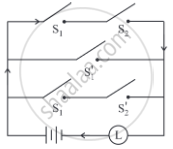Miscellaneous Exercise 1 | Q 12.2 | Page 34

Express the following circuit in the symbolic form. Prepare the switching table: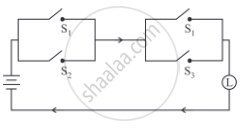Miscellaneous Exercise 1 | Q 13.1 | Page 34

Simplify the following so that the new circuit has a minimum number of switches. Also, draw the simplified circuit.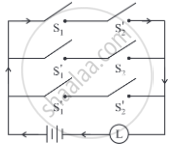Miscellaneous Exercise 1 | Q 13.2 | Page 34

Simplify the following so that the new circuit has a minimum number of switches. Also, draw the simplified circuit.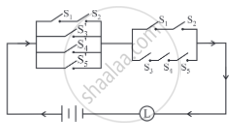Miscellaneous Exercise 1 | Q 14.1 | Page 35

Check whether the following switching circuits are logically equivalent - Justify.

(i)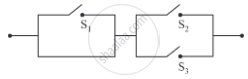(ii)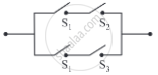Miscellaneous Exercise 1 | Q 14.2 | Page 35

Check whether the following switching circuits are logically equivalent - Justify.

(i)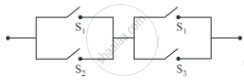(ii)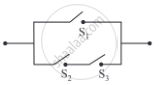Miscellaneous Exercise 1 | Q 15 | Page 35

Give alternative arrangement of the switching following circuit, has minimum switches.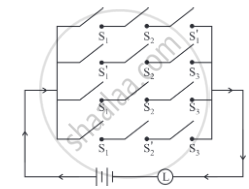Miscellaneous Exercise 1 | Q 16 | Page 35

Simplify the following so that the new circuit.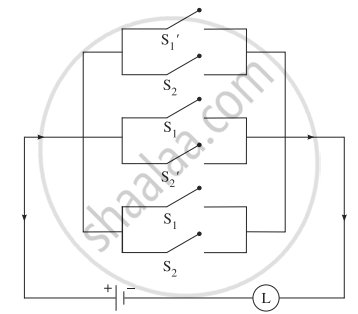Miscellaneous Exercise 1 | Q 17 | Page 35

Represent the following switching circuit in symbolic form and construct its switching table. Write your conclusion from the switching table.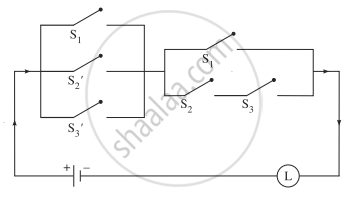Chapter 1: Mathematical Logic

Exercise 1.1Exercise 1.2Exercise 1.3Exercise 1.4Exercise 1.5Miscellaneous Exercise 1Balbharati solutions for Mathematics and Statistics 1 (Arts and Science) 12th Standard HSC Maharashtra State Board chapter 1 - Mathematical Logic

Balbharati solutions for Mathematics and Statistics 1 (Arts and Science) 12th Standard HSC Maharashtra State Board chapter 1 (Mathematical Logic) include all questions with solution and detail explanation. This will clear students doubts about any question and improve application skills while preparing for board exams. The detailed, step-by-step solutions will help you understand the concepts better and clear your confusions, if any. Shaalaa.com has the Maharashtra State Board Mathematics and Statistics 1 (Arts and Science) 12th Standard HSC Maharashtra State Board solutions in a manner that help students grasp basic concepts better and faster.

Further, we at Shaalaa.com provide such solutions so that students can prepare for written exams. Balbharati textbook solutions can be a core help for self-study and acts as a perfect self-help guidance for students.

Concepts covered in Mathematics and Statistics 1 (Arts and Science) 12th Standard HSC Maharashtra State Board chapter 1 Mathematical Logic are Concept of Statements, Truth Value of Statement, Logical Connective, Simple and Compound Statements, Statement Patterns and Logical Equivalence, Tautology, Contradiction, and Contingency, Duality, Quantifier and Quantified Statements in Logic, Negations of Compound Statements, Converse, Inverse, and Contrapositive, Algebra of Statements, Application of Logic to Switching Circuits.

Using Balbharati 12th Board Exam solutions Mathematical Logic exercise by students are an easy way to prepare for the exams, as they involve solutions arranged chapter-wise also page wise. The questions involved in Balbharati Solutions are important questions that can be asked in the final exam. Maximum students of Maharashtra State Board 12th Board Exam prefer Balbharati Textbook Solutions to score more in exam.

Get the free view of chapter 1 Mathematical Logic 12th Board Exam extra questions for Mathematics and Statistics 1 (Arts and Science) 12th Standard HSC Maharashtra State Board and can use Shaalaa.com to keep it handy for your exam preparation